YOLO 人脸识别算法以及应用 (1)

4.1 什么是人脸识别？（What is face recognition?）

4.2 One-Shot学习（One-shot learning）

4.3 Siamese 网络（Siamese network）

4.4 Triplet 损失（Triplet 损失）

4.5 面部验证与二分类（Face verication and binary classication）

4.6 什么是神经风格转换？（What is neural style transfer?）

4.7 什么是深度卷积网络？（What are deep ConvNets learning?）

4.8 代价函数（Cost function）

4.9 内容代价函数（Content cost function）

4.10 风格代价函数（Style cost function）

4.11 一维到三维推广（1D and 3D generalizations of models）

4.1 什么是人脸识别？（What is face recognition?）

（以下内容为演示视频内容）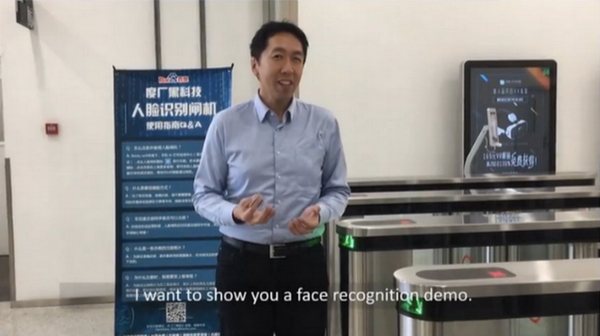NG**），不需要工卡，我就能通过了。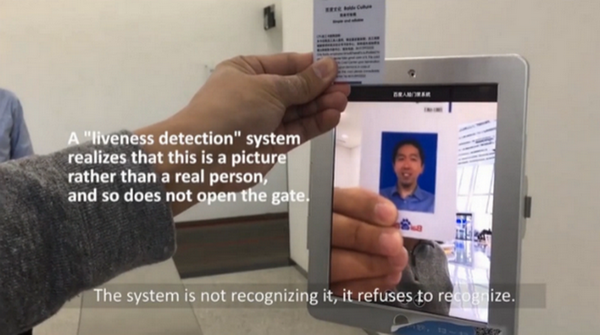（林元庆语：我将尝试用Andrew的工卡骗过机器，看看发生什么，系统不会识别，系统拒绝识别。现在我要用我自己的脸，（系统语音：“欢迎您”）（林元庆顺利通过））

#视频结束

verification**）和人脸识别（face recognition）。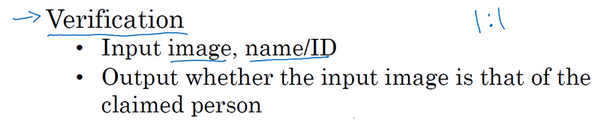）），为什么呢？假设你有一个验证系统，准确率是99%，还可以。但是现在，假设在识别系统中，[$k=100$$k=100$]
，如果你把这个验证系统应用在100个人身上，人脸识别上，你犯错的机会就是100倍了。如果每个人犯错的概率是1%，如果你有一个上百人的数据库，如果你想得到一个可接受的识别误差，你要构造一个验证系统，其准确率为99.9%或者更高，然后才可以在100人的数据库上运行，而保证有很大几率不出错。事实上，如果我们有一个100人的数据库，正确率可能需要远大于99%，才能得到很好的效果。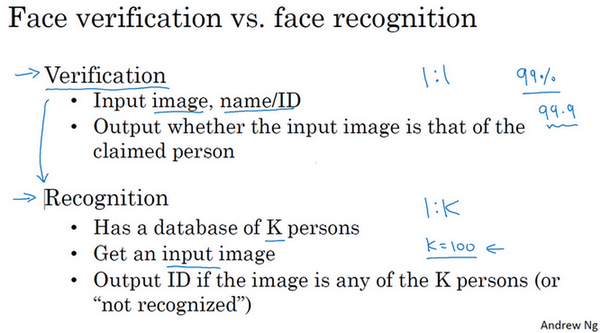learning problem**）问题。让我们看下一个视频，什么是一次学习问题。

4.2 One-Shot学习（One-shot learning）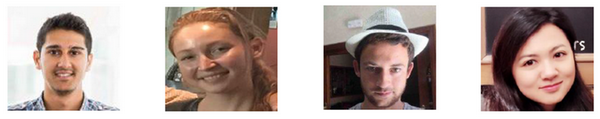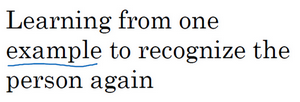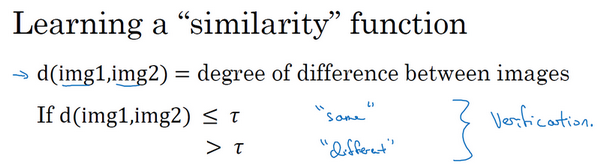，它以两张图片作为输入，然后输出这两张图片的差异值。如果你放进同一个人的两张照片，你希望它能输出一个很小的值，如果放进两个长相差别很大的人的照片，它就输出一个很大的值。所以在识别过程中，如果这两张图片的差异值小于某个阈值[$\tau$$τ$]
，它是一个超参数，那么这时就能预测这两张图片是同一个人，如果差异值大于τ，就能预测这是不同的两个人，这就是解决人脸验证问题的一个可行办法。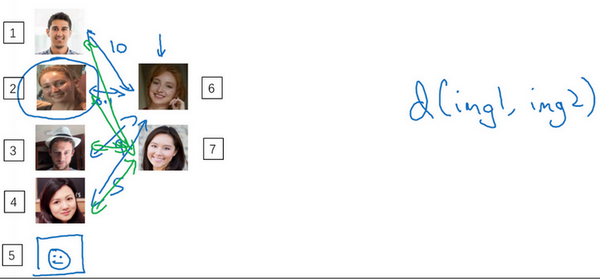，通过输入一对图片，它将会告诉你这两张图片是否是同一个人。如果之后有新人加入了你的团队（编号5），你只需将他的照片加入你的数据库，系统依然能照常工作。

4.3 Siamese 网络（Siamese network）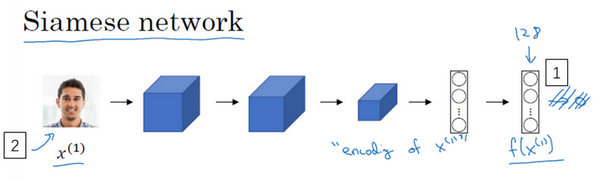，然后通过一些列卷积，池化和全连接层，最终得到这样的特征向量（编号1）。有时这个会被送进softmax单元来做分类，但在这个视频里我们不会这么做。我们关注的重点是这个向量（编号1），加如它有128个数，它是由网络深层的全连接层计算出来的，我要给这128个数命个名字，把它叫做[$f\left({x}^{\left(1\right)}\right)$$f(x^{(1)})$]
。你可以把[$f\left({x}^{\left(1\right)}\right)$$f(x^{(1)})$]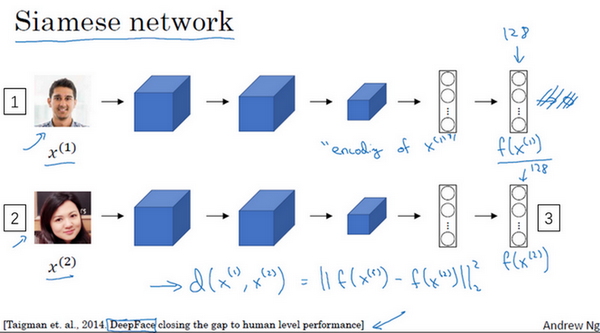。这里我用[${x}^{\left(1\right)}$$x^{(1)}$]Taigman**，Ming YangMarc’ Aurelio Ranzato，**Lior
Wolf**的这篇论文，他们开发的系统叫做DeepFace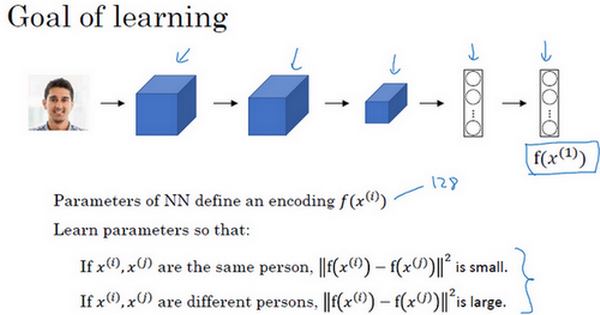4.4 Triplet 损失（Triplet 损失）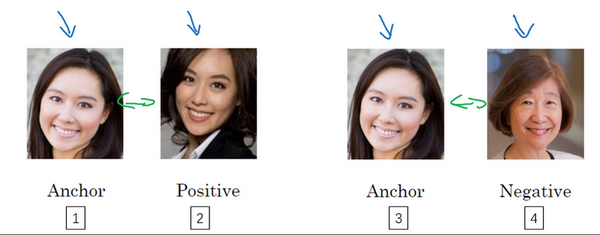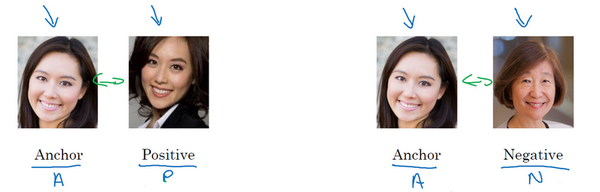，你希望这个数值很小，准确地说，你想让它小于等$f\left(A\right)$$f(A)$
$f\left(P\right)$$f(P)$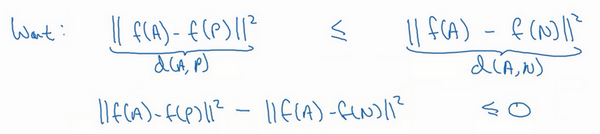[$||f\left(A\right)-f\left(P\right)|{|}^{2}<=||f\left(A\right)-f\left(N\right)|{|}^{2}$$||f(A)-f(P)||^2<=||f(A)-f(N)||^2$]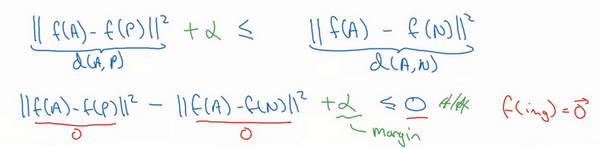（即[$||f\left(A\right)-f\left(P\right)|{|}^{2}-||f\left(A\right)-f\left(N\right)|{|}^{2}+\alpha <=0$$||f(A)-f(P)||^2-||f(A)-f(N)||^2+α<=0$]），而不是把-α

(SVM)

）也修改一下，加上这个间隔参数。

，如果 anchornegative图片的[$d$$d$]
，即[$dA,N$$d{A,N}$]

，超参数α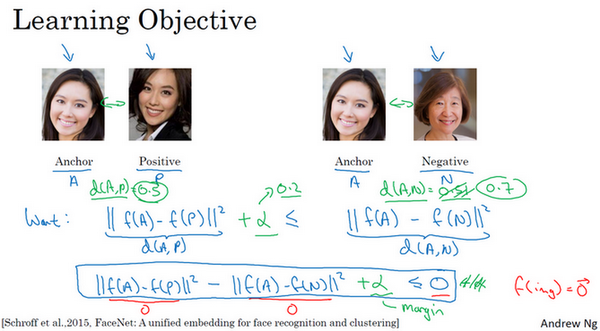，即anchor样本、positive样本和negative样本，其中positive图片和anchor图片是同一个人，但是negative图片和anchor不是同一个人。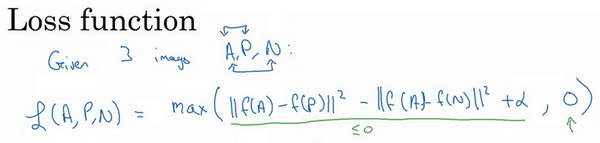[$L\left(A,N,P\right)=max\left(||f\left(A\right)-f\left(P\right)|{|}^{2}-||f\left(A\right)-f\left(N\right)|{|}^{2}+\alpha ,0\right)$$L(A,N,P)=max(||f(A)-f(P)||^2-||f(A)-f(N)||^2+α,0)$]

，那么损失函数就是0。只要你能使画绿色下划线部分小于等于0，只要你能达到这个目标，那么这个例子的损失就是0。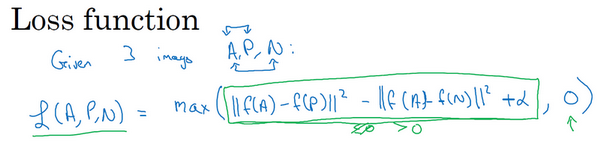，然后你取它们的最大值，最终你会得到绿色下划线部分（即[$||f\left(A\right)-f\left(P\right)|{|}^{2}-||f\left(A\right)-f\left(N\right)|{|}^{2}+\alpha$$||f(A)-f(P)||^2-||f(A)-f(N)||^2+α$]
）是最大值，这样你会得到一个正的损失值。通过最小化这个损失函数达到的效果就是使这部分[$||f\left(A\right)-f\left(P\right)|{|}^{2}-||f\left(A\right)-f\left(N\right)|{|}^{2}+\alpha <=0$$||f(A)-f(P)||^2-||f(A)-f(N)||^2+α<=0$]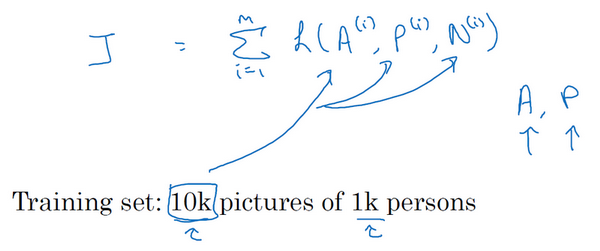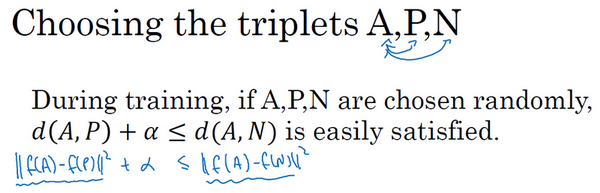、P和N，遵守A和P是同一个人，而A和N

。但是如果A

，这样网络并不能从中学到什么。、P

），或者使左边这个式子（$d\left(A,P\right)$$d(A,P)$
）变小，这样左右两边至少有一个α

Dmitry Kalenichenko, **James
Philbin**，他们建立了这个叫做FaceNet的系统，我视频里许多的观点都是来自于他们的工作。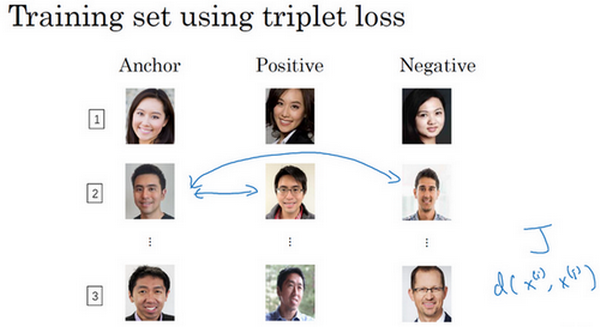4.5 面部验证与二分类（Face verification and binary classification）

**Triplet
loss**是一个学习人脸识别卷积网络参数的好方法，还有其他学习参数的方法，让我们看看如何将人脸识别当成一个二分类问题。loss**的方法。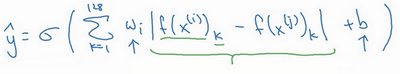），你可以利用编码之间的不同。
$y=\sigma \left(\sum _{k=0}^{128}{w}_{i}|f\left({x}^{\left(i\right)}{\right)}_{k}-f\left({x}^{\left(j\right)}{\right)}_{k}|+b\right)$$y=\sigma(\sum_{k=0}^{128}w_i|f(x^{(i)})_k-f(x^{(j)})_k|+b)$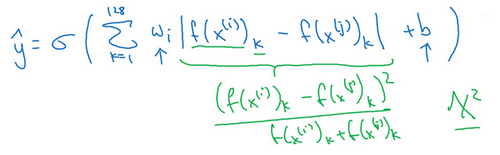），比如说，公式可以是$\frac{\left(|f\left({x}^{\left(i\right)}{\right)}_{k}-f\left({x}^{\left(j\right)}{\right)}_{k}|{\right)}^{2}}{|f\left({x}^{\left(i\right)}{\right)}_{k}+f\left({x}^{\left(j\right)}{\right)}_{k}|}$$\frac{(|f(x^{(i)})_k-f(x^{(j)})_k|)^2}{|f(x^{(i)})_k+f(x^{(j)})_k|}$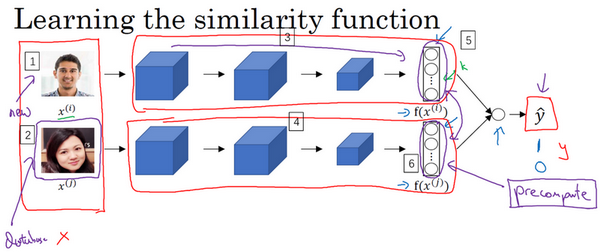（编号1、2），输出[]{.MathJax_Preview}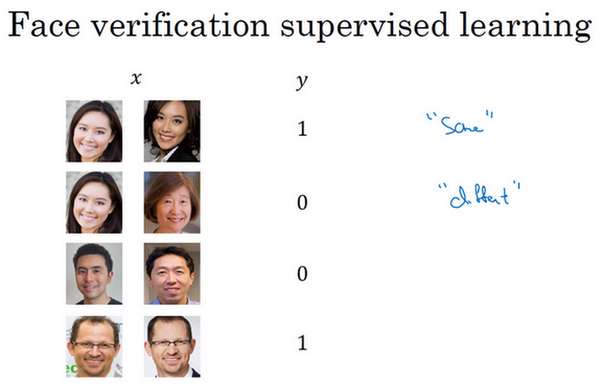4.6 什么是神经风格转换？（What is neural style transfer?）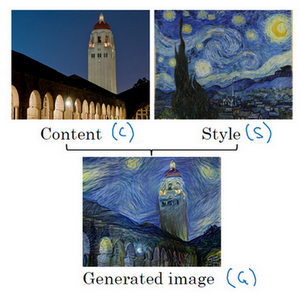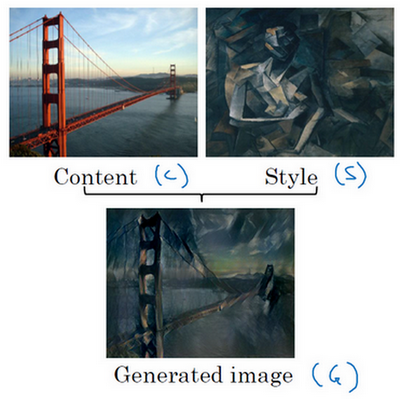Johnson**制作，在下面几个视频中你将学到如何自己生成这样的图片。

4.7 什么是深度卷积网络？（What are deep ConvNets learning?）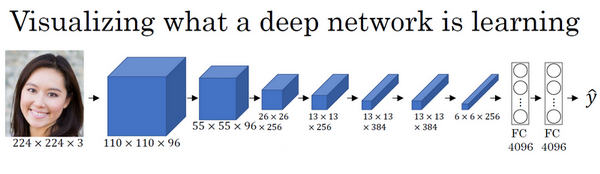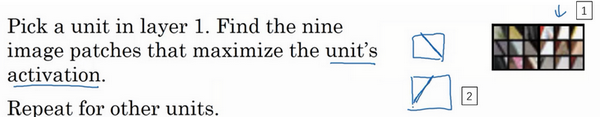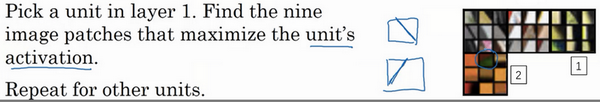Fergus**的这篇论文，题目是《可视化理解卷积神经网络》，我会使用一种更简单的方法来可视化神经网络隐藏单元的计算内容。如果你读过他们的论文，他们提出了一些更复杂的方式来可视化卷积神经网络的计算。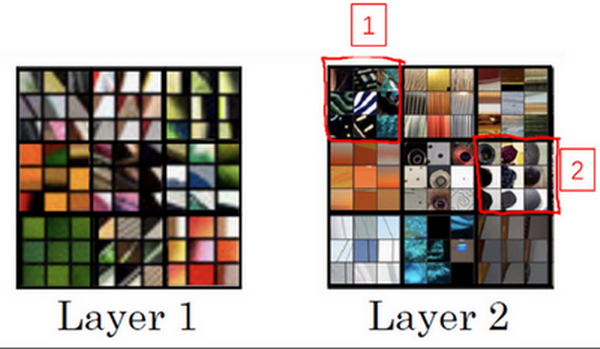1**所示图片）是之前第一层得到的，这个（**Layer
2**所示图片）是可视化的第2层中最大程度激活的9个隐藏单元。我想解释一下这个可视化，这是（编号2所示）使一个隐藏单元最大激活的9个图片块，每一个组合，这是另一组（编号2），使得一个隐藏单元被激活的9个图片块，这个可视化展示了第二层的9个隐藏单元，每一个又有9个图片块使得隐藏单元有较大的输出或是较大的激活。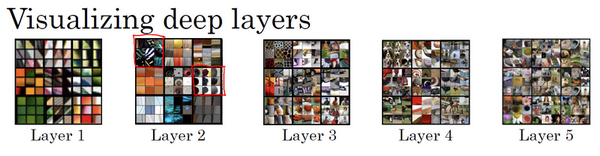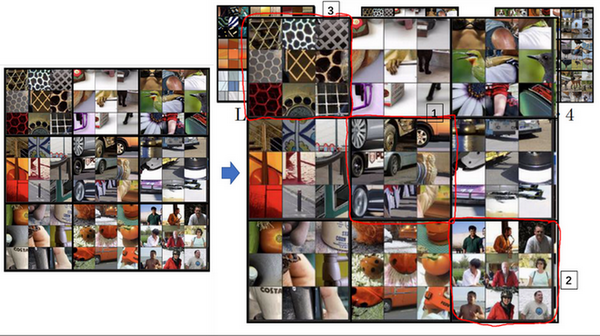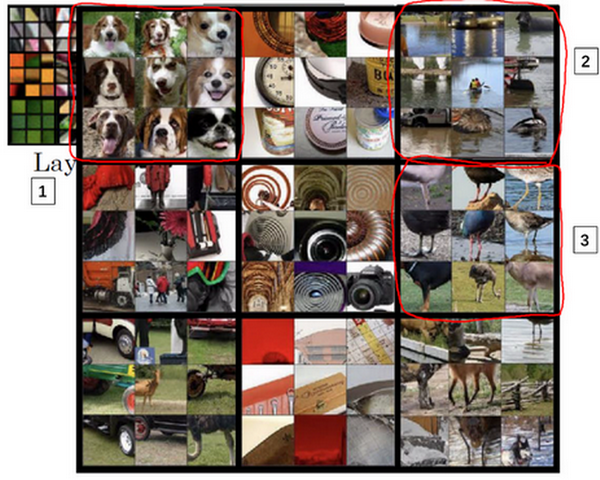4.8 代价函数（Cost function）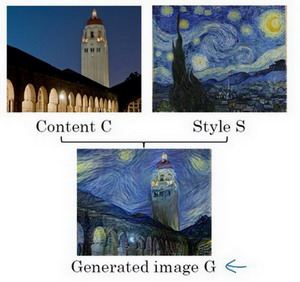，给定一个风格图片S
，而你的目标是生成一个新图片G
。为了实现神经风格迁移，你要做的是定义一个关于$J\left(G\right)$$J(G)$

，以便于生成这个图像。

${J}_{content}\left(C,G\right)$$J_{content}(C,G)$第一部分被称作内容代价，这是一个关于内容图片和生成图片的函数，它是用来度量生成图片G

${J}_{style}\left(S,G\right)$$J_{style}(S,G)$然后我们会把结果加上一个风格代价函数，也就是关于G

$J\left(G\right)=\alpha {J}_{style}\left(C,G\right)+\beta {J}_{style}\left(S,G\right)$$J(G)=\alpha J_{style}(C,G)+\beta J_{style}(S,G)$最后我们用两个超参数alphaAlexandra Ecker和**Matthias
Bethge**的这篇论文。这篇论文并不是很难读懂，如果你愿意，看完这些视频，我也非常推荐你去看看他们的论文。

，为了生成一个新图像，你接下来要做的是随机初始化生成图像G，它可能是100×100×3，可能是500×500×3，又或者是任何你想要的尺寸。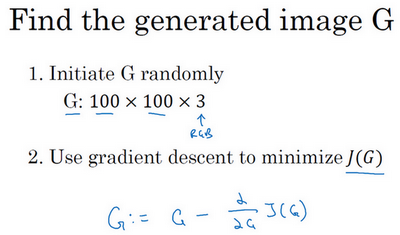。在这个步骤中，你实际上更新的是图像G的像素值，也就是100×100×3，比如RGB通道的图片。，你随机初始化的生成图像就是这张随机选取像素的白噪声图（编号3）。接下来运行梯度下降算法，最小化代价函数$J\left(G\right)$$J(G)$
，逐步处理像素，这样慢慢得到一个生成图片（编号4、5、6），越来越像用风格图片的风格画出来的内容图片。

4.9 内容代价函数（Content cost function）$J\left(G\right)=\alpha {J}_{content}\left(C,G\right)+\beta {J}_{style}\left(S,G\right)$$J(G)=\alpha J_{content}(C,G)+\beta J_{style}(S,G)$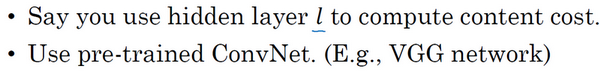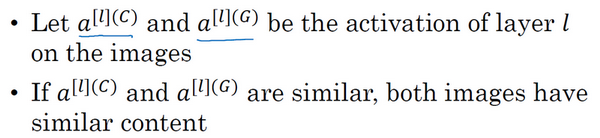${a}^{\left[l\right]\left[G\right]}$$a^{[l][G]}$
，代表这两个图片C

${J}_{content}\left(C,G\right)=\frac{1}{2}||{a}^{\left[l\right]\left[C\right]}-{a}^{\left[l\right]\left[G\right]}|{|}^{2}$$J_{content}(C,G)=\frac{1}{2}||a^{[l][C]}-a^{[l][G]}||^2$

）。，使得隐含层的激活值和你内容图像的相似。

4.10 风格代价函数（Style cost function）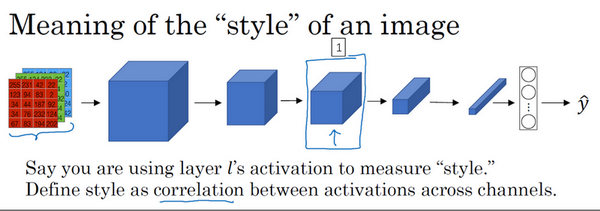（编号1），比如这一层去为图片的风格定义一个深度测量，现在我们要做的就是将图片的风格定义为l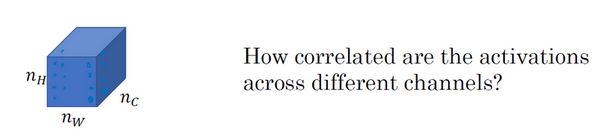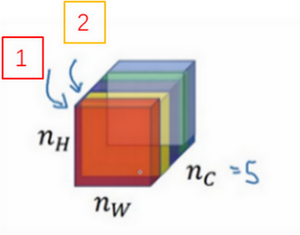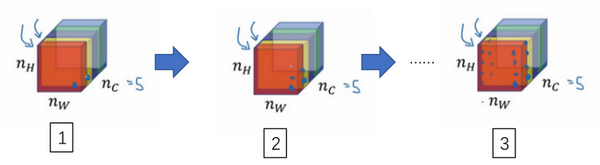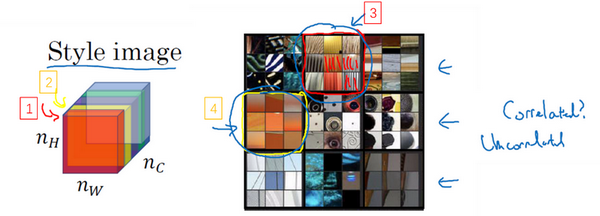Zeile**和Rob Fergus，设它为隐藏层l中(i,j,k)

（l表示层数，S表示风格图像），这是一个${n}_{c}\ast {n}_{c}$$n_c*n_c$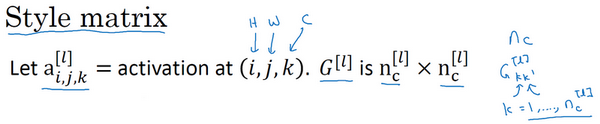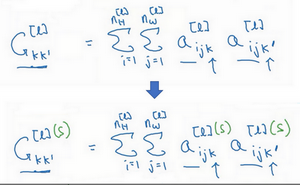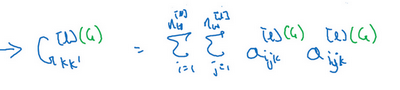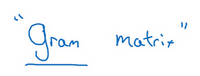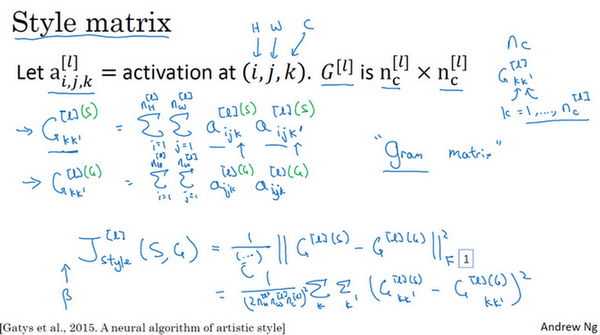Frobenius范数，编号1所示），这实际上是计算两个矩阵对应元素相减的平方的和，我们把这个式子展开，从k和k’开始作它们的差，把对应的式子写下来，然后把得到的结果都加起来，作者在这里使用了一个归一化常数，
，再在外面加一个平方，但是一般情况下你不用写这么多，一般我们只要将它乘以一个超参数$\beta$$\beta$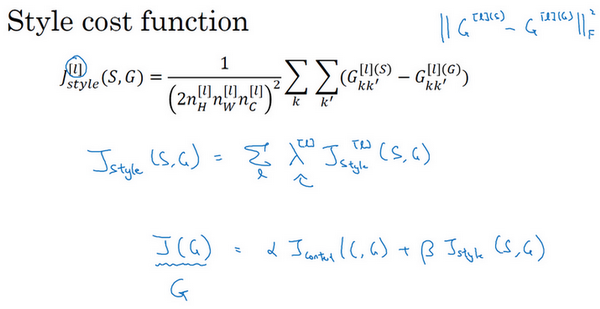，并计算J(G)